Company News

# Caverton 2019Q1: Valuation Upgrade On Future Juicier Profit, ROI

Coy: Caverton Offshore Support Group Plc (Caveton)
Current Market Price: N2.40
Year High: N3.26
Year Low: N2.40
Fair Value: N5.44
Equity Analyst: Jeariogbe Tunde Segun

Key Investment Ratios
• This report on Caverton dwells on its first quarter financial analysis for the period ended 31st March 2019, compare the same with corresponding quarter in 2018 to establish growth and other performance ratios.
• Meanwhile, the full year financials for the year ended 31st December 2018 metrics were used in the valuation analysis of each unit of CavertonOffshare.
• We have slightly stepped up our value/share for Caverton to N5.44 from our last valuation of N5.31 each.
• Judging by the soaring nature of the current first quarter financial indices over the comparable quarter of 2018, we are of the opinion that the company is beginning to reflect the dividend of its 5-year contract with the NNPC.
• We have therefore rated Caverton a Buy given the current difference between our Fair Value and the current market price.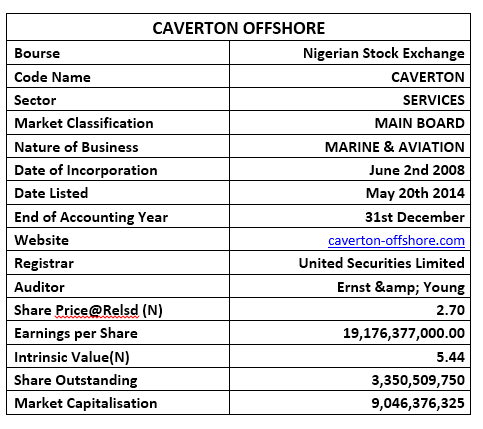Company figures
• The Turnover at the end of the first three months of 2019 stood at N8.34 billion, as against the N4.56 billion achieved during the similar quarter in 2018. Difference between the two figures stood at 82.89%
• Cost of Sales grew by 73.51% at N4.77 billion, as against the N2.79 billion posted in the 2018 first quarter
• Operating Profit is estimated at N1.68 billion, which is 89.98% above the N886.98 million in the 2018 first quarter.
• Operating Expenses through the quarter is estimated at N1.92 billion compared to the N961.40 million of 2018.
• Finance Cost rose by 12.05% at N467.34 million, as against the N417.08 million in 2018 first quarter.
• Profit before Tax (PBT) jerked up to N1.21 billion, compared to N476.52 million reported in the corresponding period of 2018.
• Profit after Tax (PAT) soared to N793.06 million, same as 170.18% over the N293.53 million earned at the end of the first three months business activities of 2018.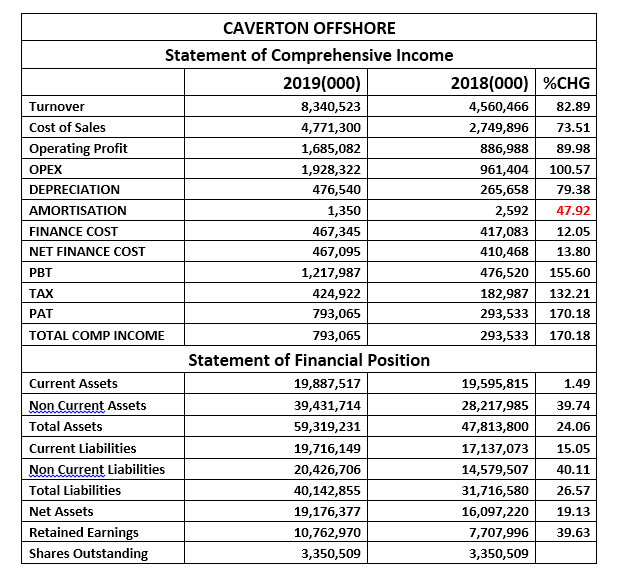• Current Assets inched north by a marginal 1.49% to N19.88 billion, as against the N19.59 billion estimate of 2018.
• Non-Current Assets, on the other hand, grew by 39.74% over a comparable period to stand at N39.43 billion against N28.21 billion
• Current Liabilities is estimated at N20.42 billion. This is 15.05% above N17.13 billion in 2018.
• Meanwhile, Non-current Liabilities appreciated by 40.11% at the present estimate of N20.42 billion, up from N14.57 billion.
• Net Assets improved to N19.17 billion from N16.09 billion in 2018 first quarter.
• Retained Earnings equally improved by 39.63% at the current estimate of N10.76 billion, compared to N7.70 billion posted in 2018 first quarter result.

Financial Strength/Solvency Ratios
• Debt Ratio is currently estimated at 0.68x higher than the 0.66x estimated from 2018 first quarter financials. This implies that Total Liabilities is same as 68% of Total Assets.
• Total Debt to Equity was estimated at 2.09x as against 1.97x estimated in the comparable quarter.
• We also tested the Equity Ratio and established that Equity is same as 32% of the Total Assets Value stated at the end of the quarter, which is fairly below the 34% ratio estimated from 2018 financial indices.
• Beta Value at the time of this report stood below unity at 0.83x, slightly below the 0.88x industry average.Profitability Ratios
• EBITDA margin estimated for the period stood at 20.20%, 3.88% above the 19.45% estimated from the corresponding quarters’ numbers.
• Pre-Tax Margin appreciated by 39.76% to 14.60% against the 10.45% estimated at the end of 2018 first quarter.
• Effective Tax Rate adjusted down by 14.05% to 53.58%, as against the 62.34% of 2018 first quarter.
• Cost of Sales figure posted at the end of the quarter is the same as 57.21% of the Turnover figure, which shows a downward marginal adjustment of 5.13% from the previous estimate of 60.30%.
• Return on Equity stood at 4.14% against 1.82% of 2018 first quarter.
• Similarly, Return on Assets soared by 117.78% to stand at 1.34% as against 0.61% of 2018 first quarter.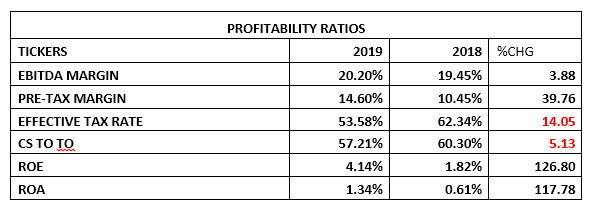Efficiency Ratios
• Operating Expenses to Turnover value is same as 23.12%, or a 9.67% growth over the 21.08% estimated from 2018 first quarter financials.
• Turnover to Total Assets Ratios stood at 14.06% as against the previous 9.54%, a 47.42% improvement in efficiency over the comparable period of 2018.
• Working Capital Ratio, which indicates a company’s effectiveness in using its working capital, was estimated at 48.67x compared to the 1.85x estimated in a similar period of 2018. This implies that the management is being extremely efficient in using the firm’s short-term assets and liabilities to support sale.
• Further testing the company efficiency, we tested the Working Capital Ratio, which confirmed that Current Assets is slightly lower than current liabilities. We also established a drop in this efficiency ratio from the previous 1.14x to 1.01x.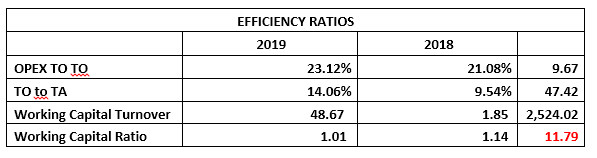Investment/Valuation Ratios
• At the end of the first three months of 2019, the management achieved earnings per share improvement to the tune of 170.18% from the previously estimated N0.09 to N0.24
• P/E-Ratio slipped by 58.36% having moved to 11.41x from 27.39x. Please understand that the reduction in this ratio is an indication that price did not follow the same momentum as growth in earnings per share.
• The said Earnings per Share is an 8.77% yield of the current market price of Caverton Offshore Plc shares on the floor of the exchange as at the time the result was made available to the investing public
• We have estimated the Book Value of each share of Caverton Offshore at N5.72, a significant improvement over the previous estimate of N4.80 and slightly above our previously estimated intrinsic value.
• Confirming the under-priced position of the stock is the Price to Book Value Ratio currently estimated below unity at 0.47x against 0.50x.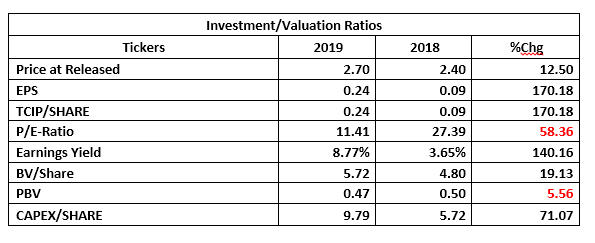Valuation
Stated in the table below are two metrics we used for valuing each unit of Caverton Offshore. We, therefore, used the average of both, following which we have fairly valued the shares at N5.44/unit and Rated it a long term Buy.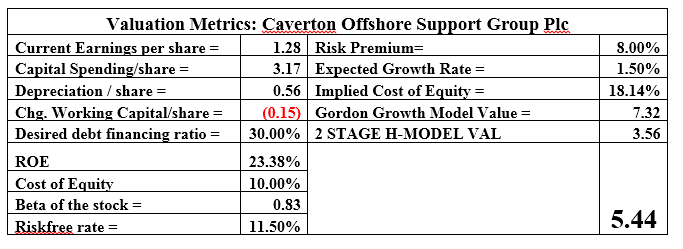Check Also
Close